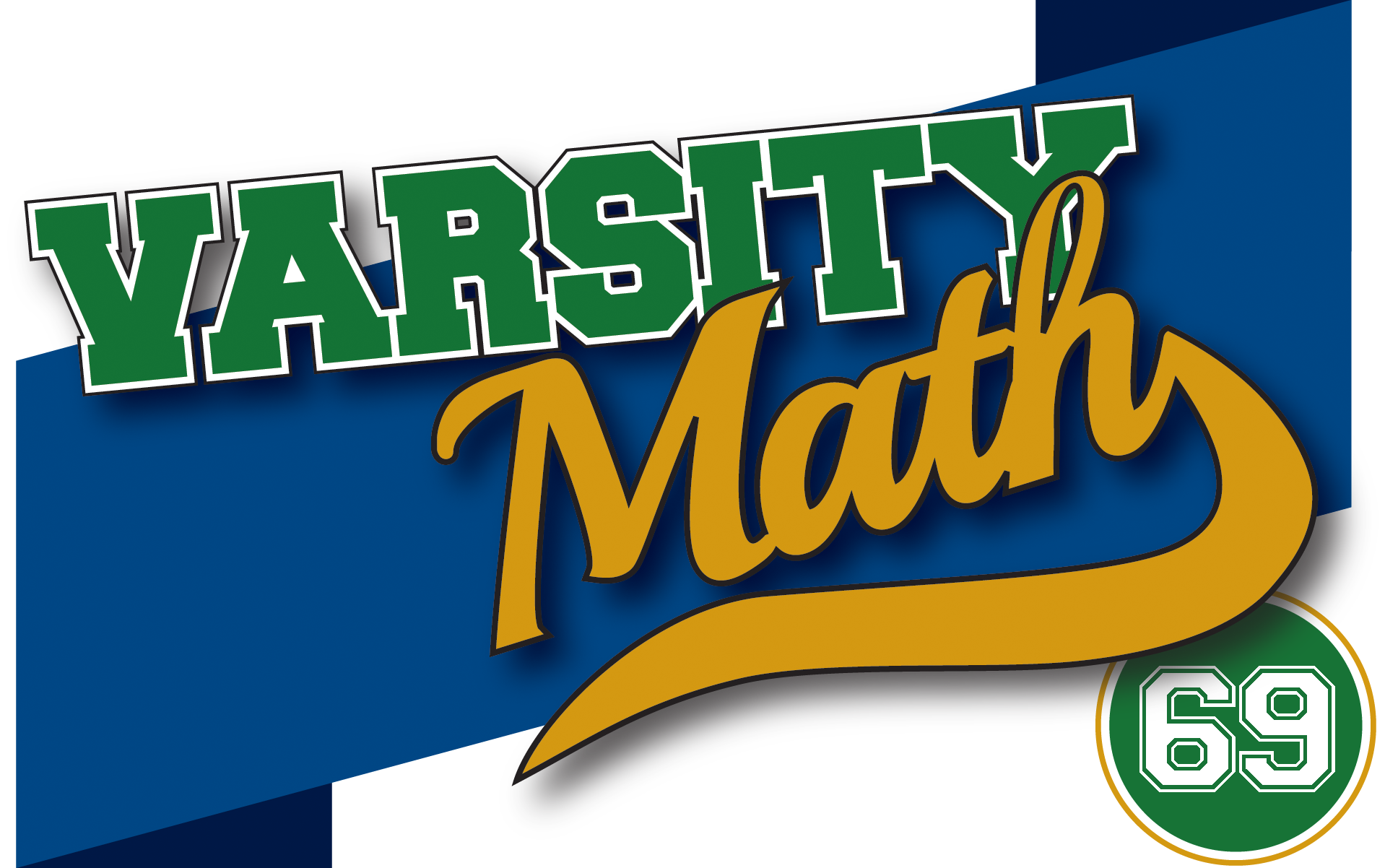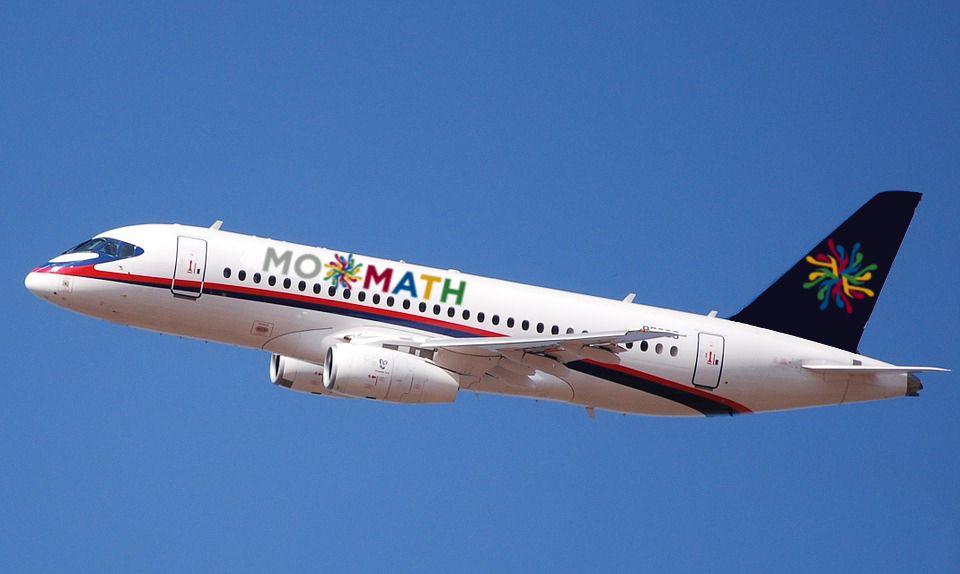## ________________

Coach Newton gets to talking with a fellow passenger on a plane, who says that a math coach “must really be good with numbers.” Coach Newton chuckles and says, “Not really, math is about much more than numbers!” But the remark does provide the inspiration for a couple of number-related problems this week.

## ________________### Not Dozens

Chris adds up all of the positive whole numbers less than 1,728 that are not integer multiples of twelve.

What sum does Chris obtain?

### Festive Factorial

To ring in the New Year, here’s a problem about the number 2017.

What is the last non-zero digit of 2017! (the factorial of 2017 = 2017 × 2016 × … × 2 × 1)?

## Solutions to week 68Snug Circle. As you can see in the diagram, there are two possible positions for circle a, centered on either point A or point A‘. However, the two positions are symmetric with respect to the y-axis, so the coordinates of point A will have the desired absolute values. The y-coordinate is easy; since radius AC is perpendicular to the x-axis and has length two, the y-coordinate of A is two. To get the x-coordinate, we need the length of horizontal line AD. But that is one leg of a right triangle with other leg BD of length 6 – 2 = 4, and hypotenuse BA of length 6 + 2 = 8. Therefore, the length of AD is √(64 – 16) = √48 = 4√3, and the desired coordinates are (4√3, 2).

Congruence Time. The minute hand on a clock makes a complete circuit of 360° in 60 minutes, so it travels at 6° per minute, and since both minute hands start pointing straight up vertically, each minute hand points 6m degrees clockwise of vertical at m minutes after midnight in Los Angeles. The hour hand makes a complete circuit in 12 hours, which is 720 minutes, and so it moves at half a degree per minute. Thus, the Los Angeles hour hand points m/2 degrees clockwise of vertical at m minutes after midnight. Since the New York clock starts with the hour hand pointing to the three, which is 90° clockwise of vertical, at that same time the New York hour hand points 90 + m/2 degrees clockwise of vertical. When they form the same angle, we must have 6mm/2 = 90 + m/2 – 6m. Solving, this is 11m = 90, which means that the two clocks make the same angle 8 2/11 minutes after midnight in Los Angeles.

## Recent Weeks

Links to all of the puzzles and solutions are on the Complete Varsity Math page.

Come back next week for answers and more puzzles.

[asciimathsf]